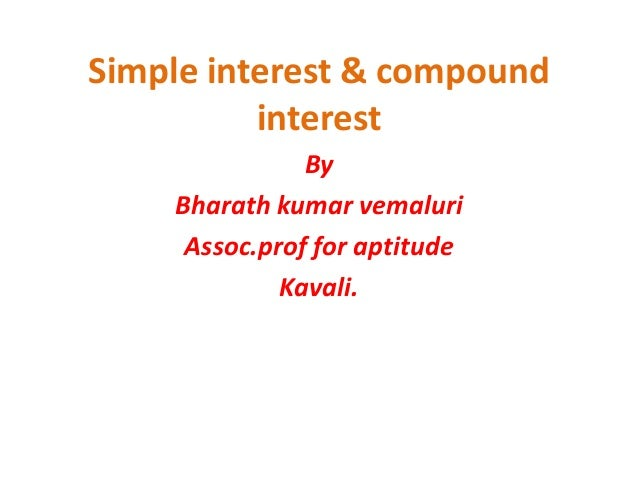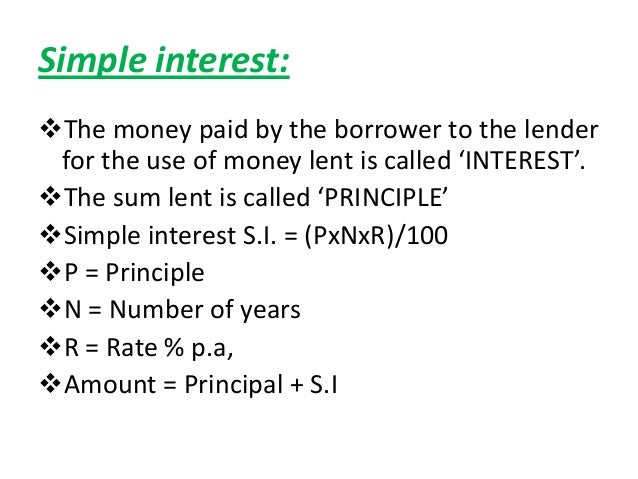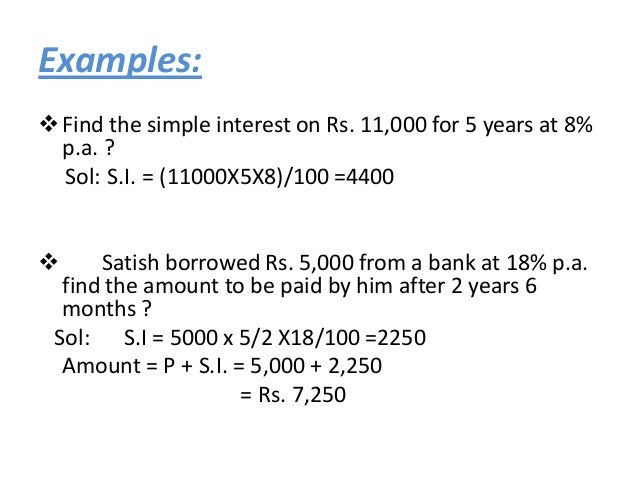Successfully reported this slideshow.Upcoming SlideShare
×

ofUpcoming SlideShare
Simple and Compound Interest
Next

Share

# Simple interest & compound interest vedio

simple interest and compound interest video is used to solve the sums in easy and quickly.

See all

See all

### Simple interest & compound interest vedio

1. 1. Simple interest & compound interest By Bharath kumar vemaluri Assoc.prof for aptitude Kavali.
2. 2. Simple interest:The money paid by the borrower to the lender for the use of money lent is called ‘INTEREST’.The sum lent is called ‘PRINCIPLE’Simple interest S.I. = (PxNxR)/100P = PrincipleN = Number of yearsR = Rate % p.a,Amount = Principal + S.I
3. 3. Examples: Find the simple interest on Rs. 11,000 for 5 years at 8% p.a. ? Sol: S.I. = (11000X5X8)/100 =4400 Satish borrowed Rs. 5,000 from a bank at 18% p.a. find the amount to be paid by him after 2 years 6 months ? Sol: S.I = 5000 x 5/2 X18/100 =2250 Amount = P + S.I. = 5,000 + 2,250 = Rs. 7,250
4. 4. Mr. Govind lent Rs. 1800 to Mr. Khan for 3 years and Rs. 3300 toMr. Mukund for 2 years and he received Rs. 480 as simple interest inall. What is the rate of interest per annum?Sol: S.I. obtained by Mr. Govind = (1800X3XR)/100 + (3300X2XR)/100 = 54 R + 66 R = 120 R = Rs. 480 R = 4% p.a.A certain sum of money is invested by Mr. Gopal at 7% p.a. after 4years. However he would get Rs. 520 more simple interest, if heinvest the same sum a t6% p.a. for 9 years. What is the sum?Sol: The difference between S.I. obtained in two cases is Rs.520 ( PX9X6)/100 – (PX4X7)/100= 520 54P-28P=52000 26P=52000 p=52000/26 = 2000
5. 5.  In how many years will a sum of money become fivetimes at 20% p.a. simple interest?Sol: Here Amount = 5P…………….(A=P+4P=5P) S.I. = 4P 4P = (PXNX20)/100 => N = 20 years
6. 6. Note: in S.I the interest remains constant or is same forevery year. in S.I the interest percentages over the year canbe added. in C.I the interest percentages over the years areto be multiplied individually.
7. 7. Compound interest: Calculate the C.I 0n 10000 at 20% per annum after 2 years. The interest being compounded annually? soln: p=10000 T=2 years R%=20% amount=10000X1.2X1.2=12400 C.I=12400-10000 = 2400
8. 8. Calculate the C.I on 10000 at 10% per annum after 1.5years the interest being compounded halfyearly?Soln: p= 10000 T=1.5 years (6 months+ 6 months+ 6 months) R%=10% ,so for six months 5%Amount= 10000X1.05x1.05X1.05=11576.25C.I= amount-principle=11576.25-10000=1576.25. Arun deposited 8000 in HDFC bank which pays him 12%per annum for 12 months . the interest beingcompounded quarterly . find the amount?Soln: principle=8000 Time =1 year (3m+3m+3m+3m) quarter= 3 months. rate= 12% ,so 3% for every quarter amount= 8000X1.03X1.03X1.03X1.03 =9004.07
9. 9. The difference between C.I & S.I on a certain sum ofmoney after 3 years,20% per annum is 2560. find the sum?soln: p= y T= 3 years R=20%Difference between c.i and s.i is 2560,1.2X1.2X1.2X Y – 1.6 y=25601.728y-1.6y=25600.128y= 2560 y=20000/-(sum)
10. 10.  A sum of money becomes 4 times in 10 years . in how many yearswill it become 64 times on C.I? soln: *4 *4 *4 X…………………..4X……………………16X…………………..64X 10 yrs 10 yrs 10 yrs so 30 years.
•#### UsmanAbubakar27

Jul. 17, 2021
•#### SrajaldeepShukla

Feb. 11, 2021
•#### EsakkiAmmal1

Jan. 16, 2020

Total views

1,771

On Slideshare

0

From embeds

0

Number of embeds

4

0

Shares

0